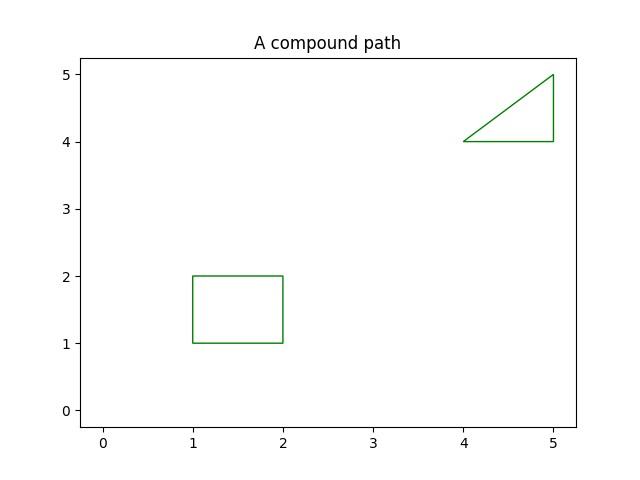# Compound path¶

Make a compound path -- in this case two simple polygons, a rectangle and a triangle. Use CLOSEPOLY and MOVETO for the different parts of the compound path

from matplotlib.path import Path
from matplotlib.patches import PathPatch
import matplotlib.pyplot as plt

vertices = []
codes = []

codes = [Path.MOVETO] + [Path.LINETO]*3 + [Path.CLOSEPOLY]
vertices = [(1, 1), (1, 2), (2, 2), (2, 1), (0, 0)]

codes += [Path.MOVETO] + [Path.LINETO]*2 + [Path.CLOSEPOLY]
vertices += [(4, 4), (5, 5), (5, 4), (0, 0)]

path = Path(vertices, codes)

pathpatch = PathPatch(path, facecolor='None', edgecolor='green')

fig, ax = plt.subplots()